#Function Repository Resource:

# SetPartitions

Give all possible ways to partition a set into blocks, ignoring the order of blocks and order within blocks

Contributed by: Wolfram Staff
 ResourceFunction["SetPartitions"][set] returns the list of set partitions of set. ResourceFunction["SetPartitions"][n] returns the list of set partitions of {1,2,…, n}.

## Details and Options

A set partition of a set S is an unordered set of non-empty disjoint subsets of S (called blocks) whose union is S.
Both the order of the blocks and the order within each block are ignored.

## Examples

### Basic Examples

There are five set partitions of a three-element set:

 In:=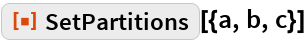Out=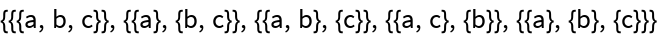In:=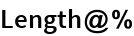Out=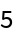The number of set partitions of a set with n elements is given by the nth Bell number:

 In:=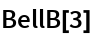Out=### Scope

Here is a compact way to see the blocks:

 In:=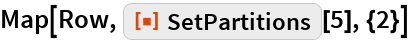Out=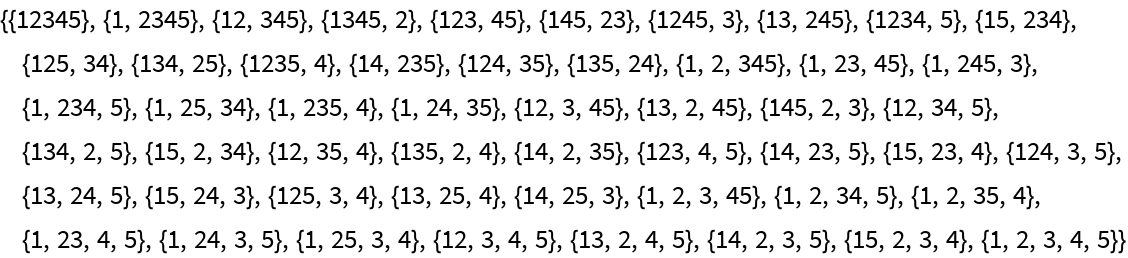## Requirements

Wolfram Language 11.3 (March 2018) or above